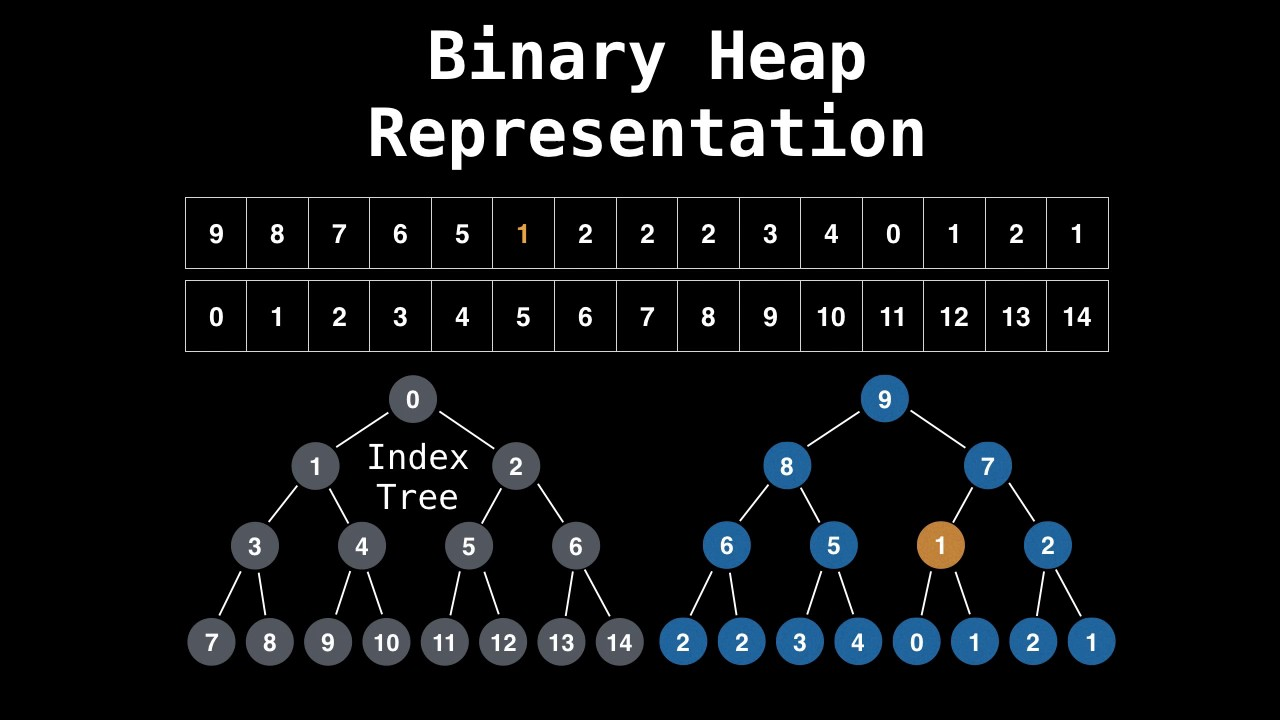Priority Queue: A data structure implementing a set S of elements, each associated with a key, supporting the following operations:

Heap:

1. implementation of a priority queue
2. An array, visualized as a nearly complete binary tree
3. Max Heap Property: The key of a node is >= than the keys of its children (Min Heap defined analogously)root of tree: first element in the array, corresponding to i = 0
parent(i) = i / 2: returns index of node’s parent
lef(i) = 2i: returns index of node’s left child
right(i) = 2i + 1: returns index of node’s right child

Heap Operator:

1. build_max_heap: produce a max_heap from an unorderred array
2. max_heapify: correct a single violation of the heap property in a subtree at its root
3. insert, extract_max, heapsort

Observe:
1. Max-Heapify take O(1) for nodes that are on level above the leaves, and in general O(l) time for nodes that are n levels above the leaves.

2. n / 4 nodes with level 1, n / 8 with level 2, …… 1 node lg(n) level

Total amount of work in the for loop is `n/4(1c) + n/8(2c) + n/16(3c) + ... + 1(lgn)`
Set n/2 = 2^k, so the formula will translate to:
`c2^k(1/2^0 + 2/2^1 + 3/2^2 + ... + (k+1)/2^k)`
Notice: `(1/2^0 + 2/2^1 + 3/2^2 + ... + (k+1)/2^k)` is a constant, which is less than 3
So the time complexity is θ(n)

Heap Sort 伪代码：